Module over a commutative unital ring

Contents

This article is about a basic definition in commutative algebra. View a complete list of basic definitions in commutative algebra

Definition

Let$R$ be a commutative unital ring. A module over$R$ is an Abelian group$M$ along with a map$.: R \times M \to M$ such that:

•$.$ is a monoid action of the multiplicative monoid of$R$ on$M$, viz.: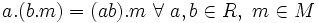$a.(b.m) = (ab).m \ \forall \ a,b \in R, \ m \in M$

and:$1.m = m \ \forall \ m \in M$

•$.$ is an additive homomorphism from$R$ (treated as an additive group) to the additive group of all functions from$M$ to itself, under pointwise addition. In symbols: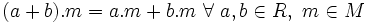$(a + b).m = a.m + b.m \ \forall \ a,b \in R, \ m \in M$

It follows that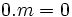$0.m = 0$ and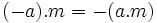$(-a).m = -(a.m)$

• The map$m \mapsto a.m$ is an endomorphism of$M$, viewed as an Abelian group.

All the above three conditions can be stated concisely as: the map$R \times M \to M$ homomorphism of unital rings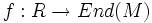$f:R \to End(M)$, where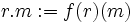$r.m := f(r)(m)$.